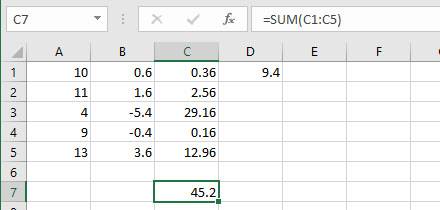Microsoft Excel is an extremely powerful spreadsheet application that is part of the Microsoft Office software package. It is installed on all Bates computers in case you don't otherwise have access to a copy.  The purpose of this site is to give you an overview of the basics of Excel.

## Cell addresses and basic formulas

A spreadsheet is comprised of individual cells, each of which has an address defined by its column letter and row number.  For example, the first cell in the upper left of a new spreadsheet has an address of A1.  Highlighting a cell by clicking with the mouse or using the arrow keys will highlight the cell.  Typing will display the contents of whatever you are typing in cell and also in the formula bar above the spreadsheet.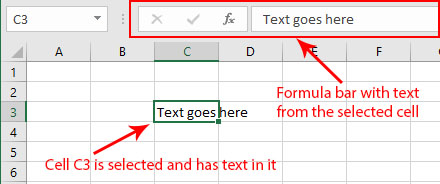In order to have excel perform a calculation instead of just displaying text, the first character in a cell must be an equal sign (=).  For example, if you type

=2+2

the cell will display the result of 4 instead of the exact text you type.  Using this approach, you can have excel function as a calculator using these basic operators

• subtract: -
• multiply: *
• divide: /
• exponent: ^ (the carat key is located over the number 6 on most standard keyboards)

You can also use parentheses to change order of operations just as you would in written math formulas.

The real power in excel comes from using the value of other cells as input into these calculations.  For example, if cell A1 contains a value of 10 (either typed directly or calculated using some formula), you could use the following formula in a different cell

=A1^2

to square that value and display 100.  If the value in A1 changes, any cell that refers to it will automatically be updated as well.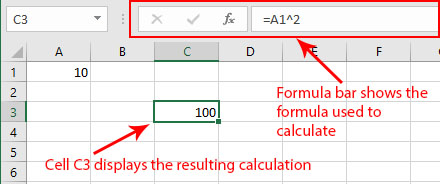Formulas can reference more than one cell. If you wanted to add the contents of two cells, A1 and B1, you could select a different cell and use the following formula

=A1+B1

Time-saving tip: Once you type the equal sign to enter formula mode, you can use the mouse or the arrow keys to select the cell you are referencing instead of typing the cell address.  Hit the Enter key when you are done typing to see the result.

## Using built-in formulas

Excel has built-in formulas to make complex calculations easier.  For example, the SUM function will add up a range of cells that you select instead of having to type the address of each cell in the range.  For example, the function

=A1+B1+C1+D1+E1

can be simplified using the sum function

=SUM(A1:E1)

to get the same result.  Most Excel functions use this format in which you specify the function right after the equal sign and then include the desired cell or range of cells in parentheses afterwards.

Time-saving tip

The easiest way to select a range is using the mouse.  To create the above formula, select a cell and type

=SUM(

and then use the mouse to highlight cells A1 to E1.  Then hit Enter.  You don't even need to add the closing parenthesis!

Some commonly used formulas for stats class include

• SUM
• AVERAGE
• MEDIAN
• MODE
• SKEW
• KURT - kurtosis
• STDEV - standard deviation
• VAR - variance
• SQRT - square root
• ABS - absolute value

If you aren't sure about the format of an Excel function, you can click on the fx button on the formula bar to open up a searchable list of excel formulas.  Not surpringly, google is also a useful tool for finding specific excel functions.

## Copying and pasting formulas

Any formula in a cell can be copied and pasted to other cells.  This greatly enhances the power of Excel's usefulness.  As an example, I might use Excel as a gradebook, in which a single row represents all of the grades for a single student for a semester.  Once I configure the (perhaps complicated) formula to use all of that information to calculate the final semester grade, I don't need to redo all of that effort for each additional student in the class.  I can simply copy and paste that formula for as many students as are needed.  In other words, creating a gradebook for 100 students is just as easy as creating one for 10 students.

Let's try a simpler example that uses examples from statistics.  Let's try two different ways to use Excel to calculate the sum of squares for a data set of five values using the following formulas: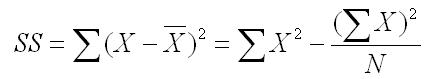We'll start with the computational formula on the right.  We'll need two sums from Excel, the sum of X values and the sum of squared X values.  Let's put our five X values in column A.  We can use column B to calculate the square of each X value.  We'll start by creating a formula in B1

=A1^2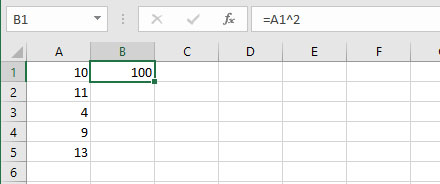Rather than adding the same type of formula in each of the cells in column B, I can use copy and paste to fill out the other 4 cells in column B with the appropriate formula.

Just click on the square in the bottom right of the selected cell B1 and drag down to include the other four cells.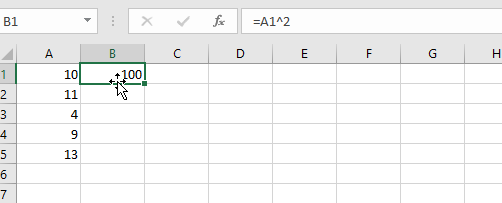Notice that the cursor changes to a plus sign when it is over the "copy and paste" section of the highlight.  If you click on the newly filled in cells, you'll see that the formula has been copied over in a RELATIVE way.  In other words, B2 references A2, B3 references A3, and so on.  The original formula in B1 references the cell one column to the left (A1), so all copied and pasted formulas will use this same spatial relationship to the new cell.

To complete the formula, we now need two sums, one for A and one for B.  We can use the SUM function to get the sum of column A, and then use the same copy and paste technique to apply that same formula to column B, as shown below.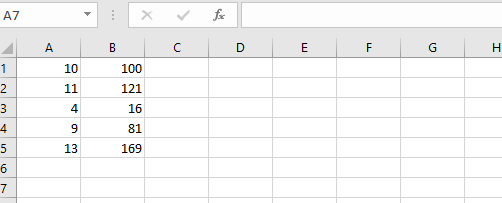## Relative vs. Absolute References

In the two copy and paste examples above, we have used relative references.  This means that the pasted formulas are adjusting the contents of the original formula to refer to cells that have the same spatial relationship as the copied formula.  In the first example, B2 refers to A2 since the copied formula in B1 refers to the cell immediately to the left (A1).

Sometimes we want the formulas to refer to the same exact cell, even when the formula is copied.  Let's use the definitional formula for sum of squares as an example.This time we'll use the middle statement of this formula which requires that we subtract the average of all X scores (X-bar) from each score to get a set of difference scores.  We then square these difference scores and add them up to get the sum of squares.

Let's start by using the AVERAGE function in cell D1 to get the average of all of the X scores in column A.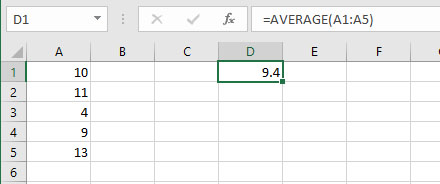I can now configure B1 to substract that average from A1.

=A1-D1

However, if I copy and paste this formula to the other cells in column B, you'll find that the A reference is correctly referring to the value in the new row, but that the D reference is also moving down each row, which is not what we want.

You can see this if you click in the formula bar for an existing formula. Excel will highlight the cells that are being used in that formula.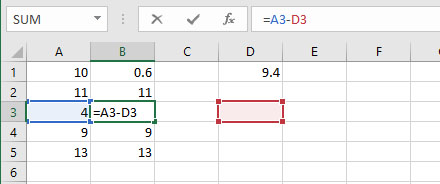Since both A1 and D1 in the original formula are relative references, they are both changing as we copy and paste the formula.

To correct this problem, we need to change D1 to be an absolute reference.  This means that any pasted formulas will always refer to cell D1, regardless of where it is pasted.  To do this, we edit the original formula in B1 and add a dollar sign (\$) in front of both the column letter and row number. (There is a shortcut key, F4, which toggles a selected cell address between relative and absolute references, putting the dollar signs in for you.) The new formula looks like this

=A1-\$D\$1

When we copy and paste this new formula to the other cells in column B, each cell will correctly refer to the data point next to them in column A and the average stored in D1.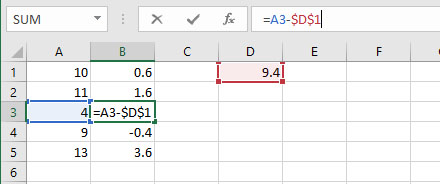We can now finish up our sum of squares calculation by using column C to square the values in column B and then add up the squared values using the SUM formula.